# Complex Puzzle

##### Age 14 to 18 Challenge Level:

Thank you to Navjot from Sherborne Qatar, Vicente from King's College, The British School of Alicante in Spain and  Jayesh, Smrithi, Alastair and Lily from Tanglin Trust School in Singapore, who all sent in correct solutions.

Some people estimated the values of $u,v$ and $w$ and then found the sums and products of these complex numbers.
For example, $u$ is approximately $0.4-0.3i$ and $v$ is approximately $0.9+0.5i$, so $u+v$ is approximately $0.4-0.3i+0.9+0.5i=1.3+0.2i$, which must be $h$.

Others used the geometry from Opening the Door, Strolling Along and Into the Wilderness. The solution below uses those ideas.

As we saw in Opening the Door, adding two complex numbers is the same as a translation. This diagram shows the real and imaginary parts of $u$ as they are added onto $v$. This is the same as putting the vector from the origin to $u$ at the point $v$: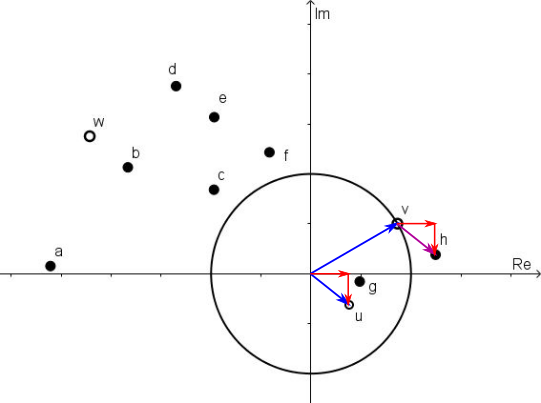So $h=v+u$, or $u+v$. In the diagram below, the vector from the origin to $v$ is placed at the point $u$, to show the addition both ways round: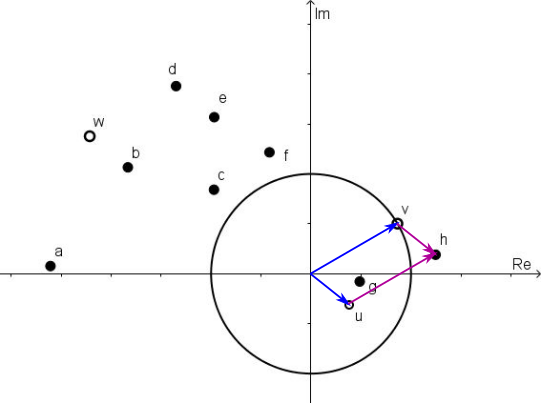The other additions can also be done in this way:

$u+w=b$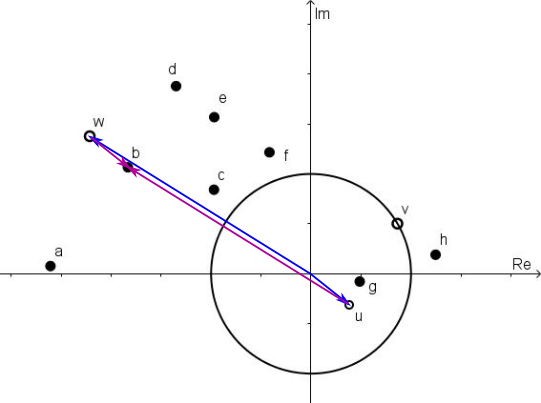$v+w=d$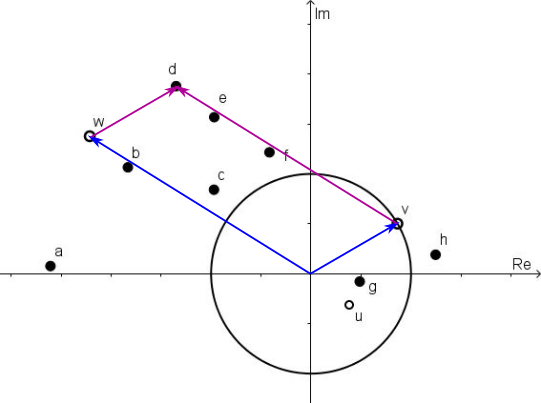$u+v+w=e$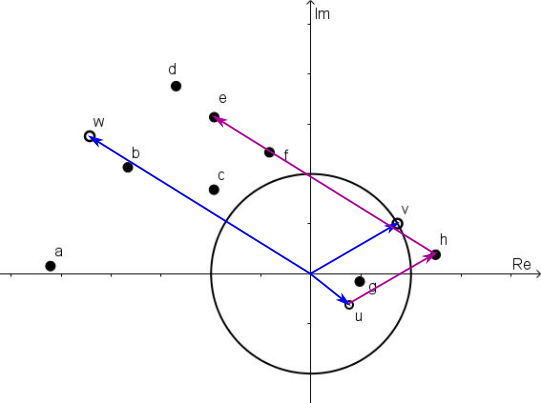Multiplication

As we saw in Into the Wilderness, multiplying a complex number by another complex number is the same as a rotation and an enlargement.

The distance from the origin to $v$ is $1$, so multiplying by $v$ will result in a rotation only (since the scale factor of the enlargement would be $1$). To find $uv$ (below), the angle from the real axis to $v$ is shown, and is placed along $u$. The dotted line shows the result of rotation through this angle. There is also a circle drawn through $u$ centred at the origin, because the distance from the origin to $uv$ is the same as the distance from the origin to $u.$ So $uv$ will lie on this circle, and go through the dotted line.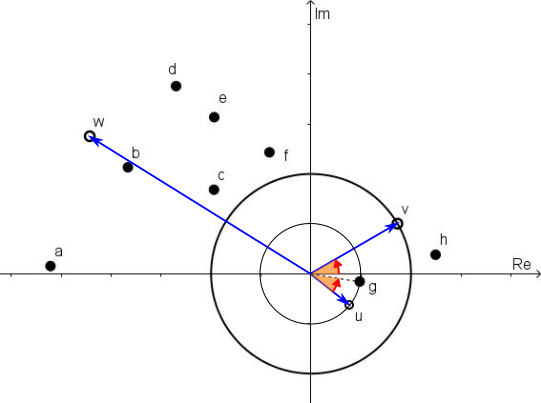$uv=g$

Similarly for $vw$: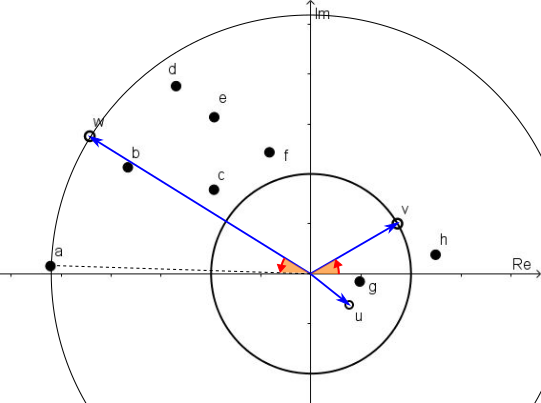$vw=a$

For the product $uw$, the scale factor of the enlargement won't be $1$. Thinking of it as multiplication by $u$, $w$ would get smaller, since the distance from the origin to $u$ is less than $1$. The rotation part is shown below, and this is sufficient to identify $uw$ as $f$: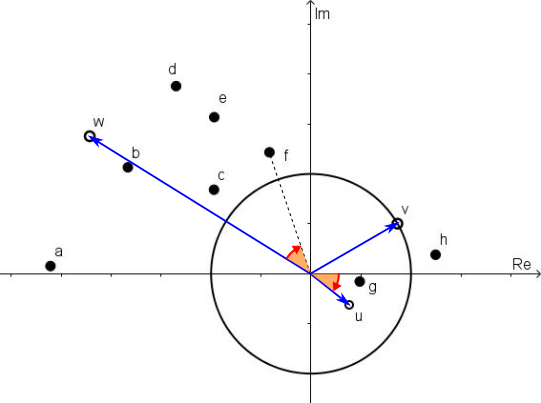To find $uvw$ (which will hopefully be $c$ as this is the only one left), we can multiply $f$ by $v$, which is a rotation again, as shown below: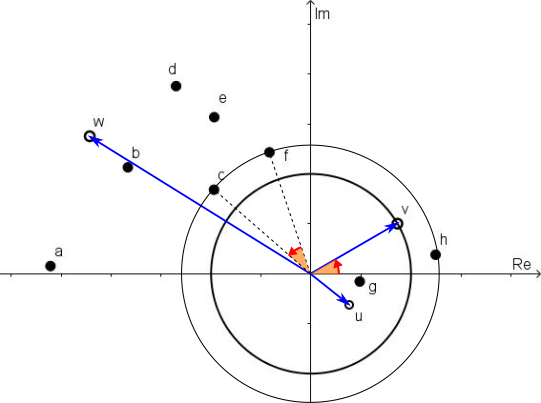So:
$u + v = h$
$u + w = b$
$v + w = d$
$u + v + w = e$
$uv = g$
$uw = f$
$vw = a$
$uvw = c$